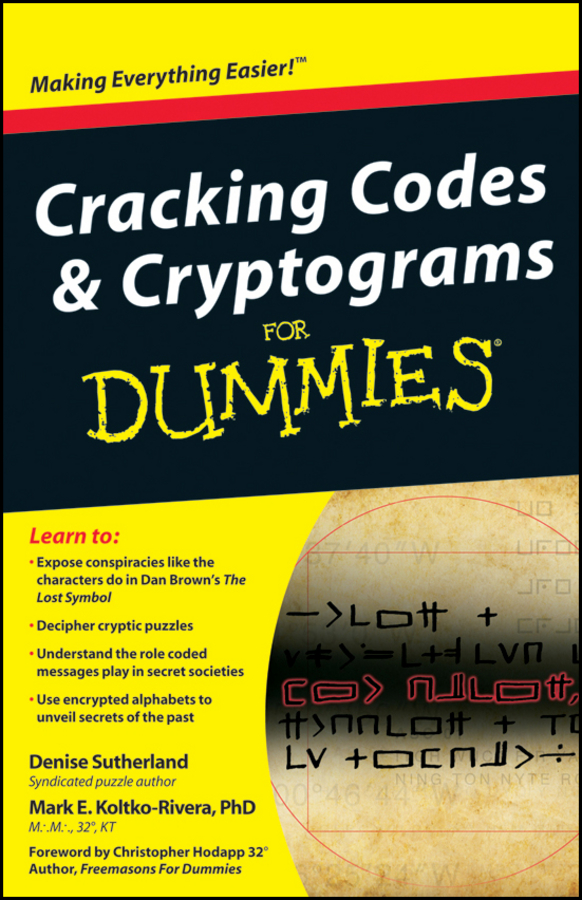##### Cracking Codes and Cryptograms For DummiesHere are some basic abbreviations that are very useful to have by your side when cracking cryptic clues! The list is concise and only focuses on the harder abbreviations. Straightforward abbreviations and simple foreign words have been omitted from this list.
 10 = X 100 = C 1,000 = M 5 = V 50 = L a follower = B about = C, CE, RE against = V (versus) alien = ET American soldier = GI ancient city = UR ancient times = BC Anglo-Saxon = AS Arabic = AR artist = RA (Royal Academy) Asian = E (Eastern) at home = IN bishop = B (chess) Bond’s boss = M book = B bridge player = N, S, E, W British = B, BR Capone = AL carbon copy = CC castle = R (rook) chapter = C or CH chief = CH Church of England = CE civil engineer = CE cross = X club/s = C (cards) Common Era = CE Cyprus = CY daughter = D degree = D, BA, MA diamond/s = D (cards) Diana = DI direction = N, S, E,W doctor = DR, MB, MD, MO dram = DR duck = O (cricketing) Elizabeth = E English = E exercise = PT or PE exist = BE fine = F (pencil), OK first = IST (1st) first class = AI (A1) fish = LING, EEL, COD etc. force = F former partner = EX France = F gas = H (hydrogen), CO (carbon monoxide), etc. George = G Georgia = GA (state), GE (country) German = G gold = AU or OR (heraldry) good = G good man = ST (saint) graduate = BA, MB Greek character or letter = PI, NU, MU, XI, etc. Guevara = CHE hard = H (pencil) heart/s = H (cards) hexadecimal = HEX honour = OBE hour = HR hug = O husband = H hush = SH I am = IM (I’m) in charge = IC instant message = IM integrated circuit = IC judge = J king = K or ER kiss = X last character = Z lawyer = BL (Bachelor of Law) letter = EF, EM, EL Liberal = L line = L (l looks like a line) loud = F (forte) midday = N (noon) model = T (Model T Ford) moment = MO monsieur = M months = MOS new = N newton = N (unit) no good = NG noon = N note = A – G, DO, RE, MI, FA, SO, LA, TI, TE, DOH, etc. nothing = O ocean = O old = O old boy = OB old city = UR old record = EP one = A, AN, I (1) operation = OP oriental = E (from the East) penny = P playing = ON (stage) point = N, S, E or W power = P present day = AD princess = DI printer’s measure = EN, EM private investigator = PI physical education = PE Public Record Office = PRO quarter = N, NE, NW, E etc queen = R, Q, or ER queen’s honour = OBE quiet = P (piano) ring = O resistance = R (unit) river = R, EXE, DEE, PO, etc. roughly = CA (circa) round = O sailor, salt = AB (able- bodied seaman) second = S second-class = B Shakespearean king = LEAR ship/s = SS short time = T single = I (1) society = S soft = P (music), B (pencil) son = S spade/s = S (cards) square = S teetotal/le = TT temperature = T thanks = TA the thing = IT times = X top class = AI (A1) translator = TR unknown = X or Y versus = V, VS very black = BB (pencil) vitamin = A, B, BI (B1), C, etc. vote = X wife = W will = LL (contraction) women’s supporter = BRA worker = ANT, BEE year = Y yours truly = I, ME